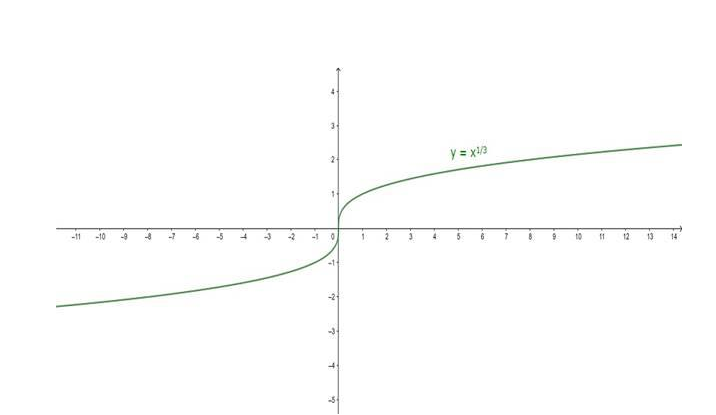# Find the domain and the range of the cube root function,

Question:

Find the domain and the range of the cube root function,

f: $R \rightarrow R: f(x)=x^{1 / 3}$ for all $x \in R$. Also, draw its graph.

Solution:

Given:

$f(x)=x^{1 / 3} \forall x \in R$

To Find: Domain and range of the given function.

Here, $f(x)=x^{1 / 3}$

The domain of the above function would be,

Domain $(f)=(-\infty, \infty)\{x \mid x \in R\}$

Because all real numbers have a cube root. There is no value of x which makes the function undefined.

Now, to find the range

Consider $f(x)=y$

Then, $y=x^{1 / 3}$

$y^{3}=x$

Since f(x) is continuous, it follows that

Range $(f)=(-\infty, \infty)\{y \mid y \in R\}$

Because for every value of y there would be a cube of that value.

Graph: